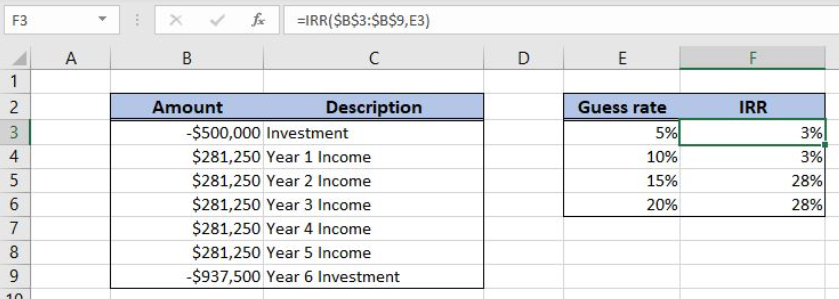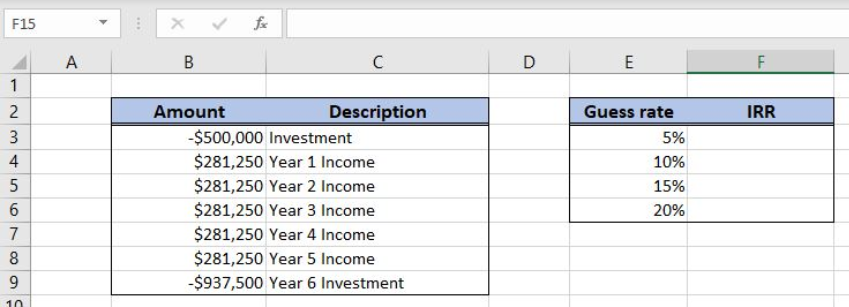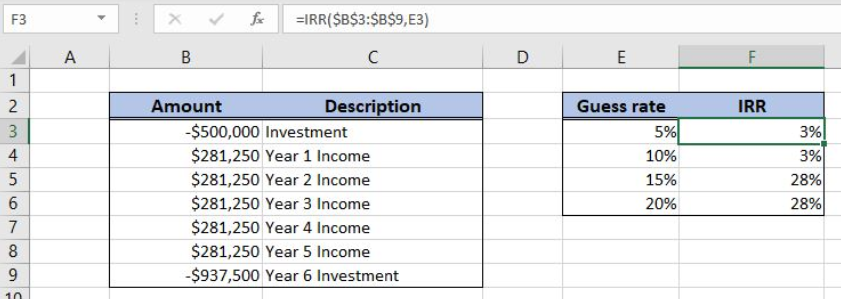Get instant live expert help with Excel or Google Sheets“My Excelchat expert helped me in less than 20 minutes, saving me what would have been 5 hours of work!”

#### Post your problem and you’ll get expert help in seconds.

Your message must be at least 40 characters
Our professional experts are available now. Your privacy is guaranteed.

# Learn How to Solve Issues with a Multiple IRR in Excel

Excel allows a user to manipulate with multiple internal rates of return using the IRR function. Multiple IRR occurs when there is a cash flow project with cash outflow. This step by step tutorial will assist all levels of Excel users to learn how to solve issues with a multiple IRR in Excel.Figure 1. The result of the multiple IRR function

## Syntax of the IRR Formula

The generic formula for the IRR function is:

`=IRR (values, [guess])`

The parameter of the IRR function is:

• values – a range of cells containing values, including initial investment and incomes. The investment must have a negative sign, as it is a cost
• [guess] – an estimated value for the expected IRR. This parameter is non-mandatory. If it’s omitted, the function will take a default value of 0.1 (=10%).

## Setting up Our Data for the IRR Function

Let’s look at the structure of the data we will use. In column B (“Amount”), we have values including initial investment, yearly incomes and cash outflow. In column C (“Description”) we have a description of every amount. In column E (“Guess rate”), we have expected IRR, while in column F, we want to calculate IRR.Figure 2. Data that we will use in the multiple IRR example

## Get an IRR of Values Using the IRR Function

In our example, we want to calculate IRR for different guess rates and see what are the results of the function.

The formula looks like:

`=IRR(\$B\$3:\$B\$9, E3)`

The parameter values is the range \$B\$3:\$B\$19. This range must be fixed, as it’s not changing when the formula is copied down the column. The parameter guess is in the cell E3.

To apply the IRR function, we need to follow these steps:

• Select cell F3 and click on it
• Insert the formula: `=IRR(\$B\$3:\$B\$9,E3)`
• Press enter
• Drag the formula down to the other cells in the column by clicking and dragging the little “+” icon at the bottom-right of the cell.Figure 3. Using the IRR function to calculate the multiple IRR

As we can see, the IRR for guess 5% and 10% is 3%, while for 15% and 20% is 28%. This means that there are two internal rates of return at which the net present value of the cash flow is equal to 0.

Most of the time, the problem you will need to solve will be more complex than a simple application of a formula or function. If you want to save hours of research and frustration, try our live Excelchat service! Our Excel Experts are available 24/7 to answer any Excel question you may have. We guarantee a connection within 30 seconds and a customized solution within 20 minutes.